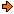WON plate143 | World!OfNumbers[ November 12, 2002 ] Palindromic Primes stretching out until... Jeff Heleen (email) I have found 3 palprimes. I don't know if you have them somewhere already on your site but here they are. If you start with a central 1 and concatenate the counting integers to either side (mirrorwise to the left),the palprimes occur at N = 31, 59 and 113,all three N values are coincidentally prime ! 1303928272...7654321234567...2728293031 = prime (105 digits) 9585756555...7654321234567...5556575859 = prime (217 digits) 311211111011...4321234...110111112113 = prime (417461 digits) My search went up to N = 999. I used Primo for the tests. Who can extend this list with a few more terms ?[ November 15, 2002 ] Jean Claude Rosa wrote that the third number had a wrong length indication 417 instead of 461. "Soit P = n... ...32123... ...n avec 99 < n < 1000 pour avoir la longueur de P j'utilise la formule suivante : longueur de P = 6 * n - 217 Si n = 113, longueur de P = 6 * 113 - 217 = 461."[ November 17, 2002 ] Jeff Heleen has another possible palprime for this plate. For N = 1277 (a prime itself) shows promise. This would yield a number with 8001 digits (assuming I have added correctly). I shall not attempt to prove it prime at this time as it would take far too much time. PDG tested this candidate with PFGW [ July 13, 2004 ] but to my surprise the outcome was that this number is composite ! In case I made a mistake perhaps someone would like to confirm that this palindrome is not a probable prime ?[ February 3, 2022 ] I, Patrick De Geest, refreshed the above search but now with the aid of a Pari/gp script. All it does is to make a list of all the candidate palindrome primes into a file "Mirror.txt". From there on that list is passed through to pfgw64 who tests all those entries for probable-prime-ness. Here is my code. ```{ x=[];y=[]; for(i=1,19999, p=digits(i); x=concat(x,p); y=(Vecrev(x)); z=fromdigits(concat(y, x[2..length(x)])); ld=x[length(x)]; if((ld==1||ld==3||ld==7||ld==9), print(i," ",z); write("C:/pari/Mirror.txt", z)); ); }``` pfgw64 "C:\pari\Mirror.txt" In the Pfgw64 folder you'll find a log file (pfgw.log) with all the 3-PRP!'s he found. Note that the candidate with N = 9999 has 77777 digits ! The mirrorleft and right number N reached now 19999 (177,777 digits) [ March 18, 2022 ] and still no fourth (probable) prime. So, if there is a fourth companion N must be greater than  31   59   113   N > 19999There is of course the similar case where we have as left part the concatenations of the descending numbers (without reversing them) or the Rsm series13_12_11_10_9_8_7_6_5_4_3_2_1 followed on the right by the mirror image of the above Rsm section 13_12_11_10_9_8_7_6_5_4_3_2_1_2_3_4_5_6_7_8_9_01_11_21_31 ```{ x=[];y=[]; for(i=1,19999, p=digits(i); x=concat(p,x); y=(Vecrev(x)); z=fromdigits(concat(x, y[2..length(y)])); ld=y[length(y)]; if((ld==1||ld==3||ld==7||ld==9), print(i," ",z); write("C:/pari/MirRev.txt", z)); ); }``` pfgw64 "C:\pari\MirRev.txt" Two primes popped up after a short run of the Pari/gp code. [ 7372717069... ...12111098765432123456789011121... ...6907172737 ] with 273 digits and [ 130129128127 ... ...1110987654321234567890111... ...721821921031 ] with 563 digits Let me now go throught the higher values and see if more primes shows up. The mirrorright and left number N reached now 19999 (177,777 digits) [ May 8, 2022 ] and still no third (probable) prime. For now I collected  73   130   N > 19999A000143Prime Curios!Prime PuzzleWikipedia 143Le nombre 143```

```

[TOP OF PAGE]

Patrick De Geest - Belgium- Short Bio - Some Pictures